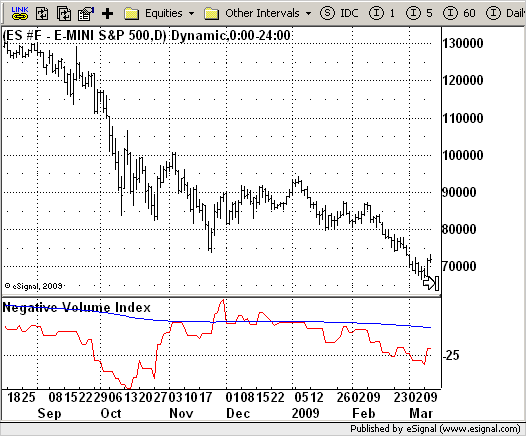# Negative Volume Index

ICE Data Services -

 NVI_Peterson.efs EFSLibrary - Discussion Board

File Name: NVI_Peterson.efs

Description:
Negative Volume Index

Formula Parameters:
EMA_Len : 255

Notes:
The theory behind the indexes is as follows: On days of increasing
volume, you can expect prices to increase, and on days of decreasing
volume, you can expect prices to decrease. This goes with the idea of
the market being in-gear and out-of-gear. Both PVI and NVI work in similar
fashions: Both are a running cumulative of values, which means you either
keep adding or subtracting price rate of change each day to the previous day`s
sum. In the case of PVI, if today`s volume is less than yesterday`s, don`t add
anything; if today`s volume is greater, then add today`s price rate of change.
For NVI, add today`s price rate of change only if today`s volume is less than
yesterday`s.`/*********************************Provided By: eSignal (Copyright c eSignal), a division of Interactive Data Corporation. 2009. All rights reserved. This sample eSignal Formula Script (EFS) is for educational purposes only and may be modified and saved under a new file name. eSignal is not responsible for the functionality once modified. eSignal reserves the right to modify and overwrite this EFS file with each new release.Description: Negative Volume Index Version: 2.0 05/28/2009 Formula Parameters: Default: EMA_Len 255 Notes: The theory behind the indexes is as follows: On days of increasing volume, you can expect prices to increase, and on days of decreasing volume, you can expect prices to decrease. This goes with the idea of the market being in-gear and out-of-gear. Both PVI and NVI work in similar fashions: Both are a running cumulative of values, which means you either keep adding or subtracting price rate of change each day to the previous day`s sum. In the case of PVI, if today`s volume is less than yesterday`s, don`t add anything; if today`s volume is greater, then add today`s price rate of change. For NVI, add today`s price rate of change only if today`s volume is less than yesterday`s. **********************************/var fpArray = new Array();var bInit = false;function preMain(){ setStudyTitle("Negative Volume Index"); setCursorLabelName("NVI",0); setDefaultBarFgColor(Color.red,0); setCursorLabelName("EMA",1); setDefaultBarFgColor(Color.blue,1); var x = 0; fpArray[x] = new FunctionParameter("EMA_Len", FunctionParameter.NUMBER); with(fpArray[x++]) { setLowerLimit(1); setDefault(255); } }var xNVI = null;var xNVI_EMA = null;function main(EMA_Len) {var nBarState = getBarState();var nNVI = 0;var nEMA = 0; if (nBarState == BARSTATE_ALLBARS) { if(EMA_Len == null) EMA_Len = 255; } if (bInit == false) { xNVI = efsInternal("Calc_NVI"); xNVI_EMA = ema(EMA_Len, xNVI); bInit = true; } nNVI = xNVI.getValue(0); nEMA = xNVI_EMA.getValue(0); if (nEMA == null) return; return new Array(nNVI, nEMA);}var bSecondInit = false;var xROC = null;var xVolume = null;function Calc_NVI() {var nRes = 0;var nRef = ref(-1); if (bSecondInit == false) { xROC = roc(1); xVolume = volume(); bSecondInit = true; } if (xROC.getValue(-1) == null) return; if(xVolume.getValue(0) < xVolume.getValue(-1)) { nRes = nRef + xROC.getValue(0); } else { nRes = nRef; } return nRes;}`